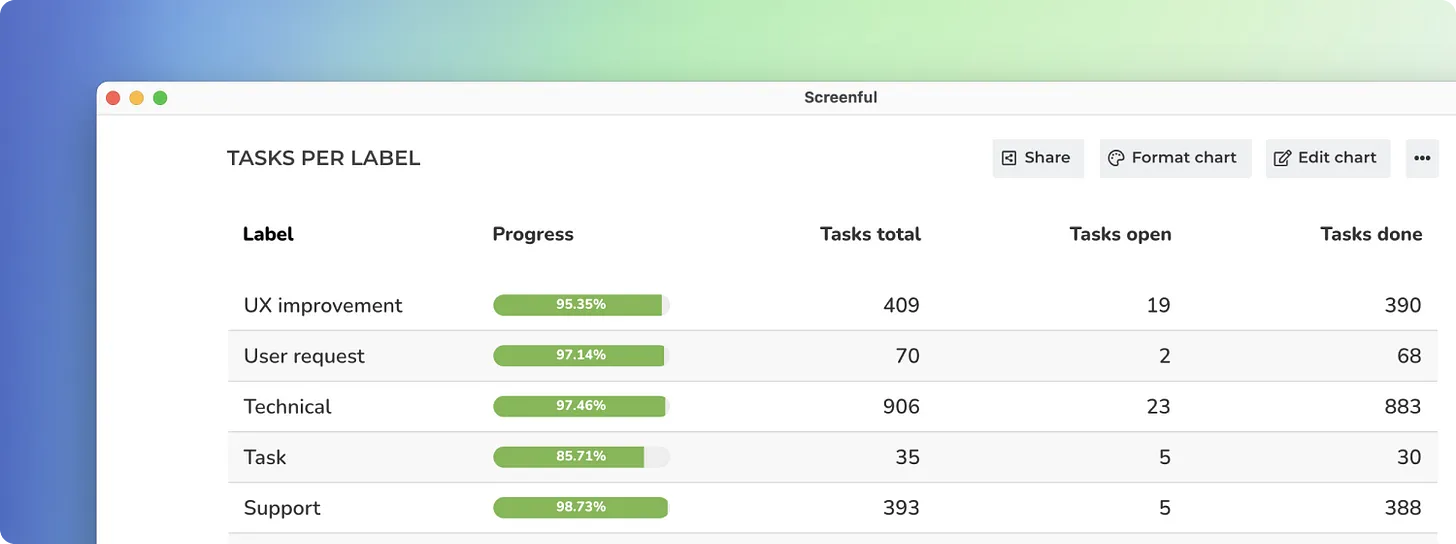# Table chart with custom formulas

### Feb 28, 2021

We have added a new chart type into the library of available custom charts. The new Table chart allows to view data in rows and columns. You can choose how your data is grouped into rows, and you can configure the columns to display exactly the information you need.

Here’s an example of a Table chart: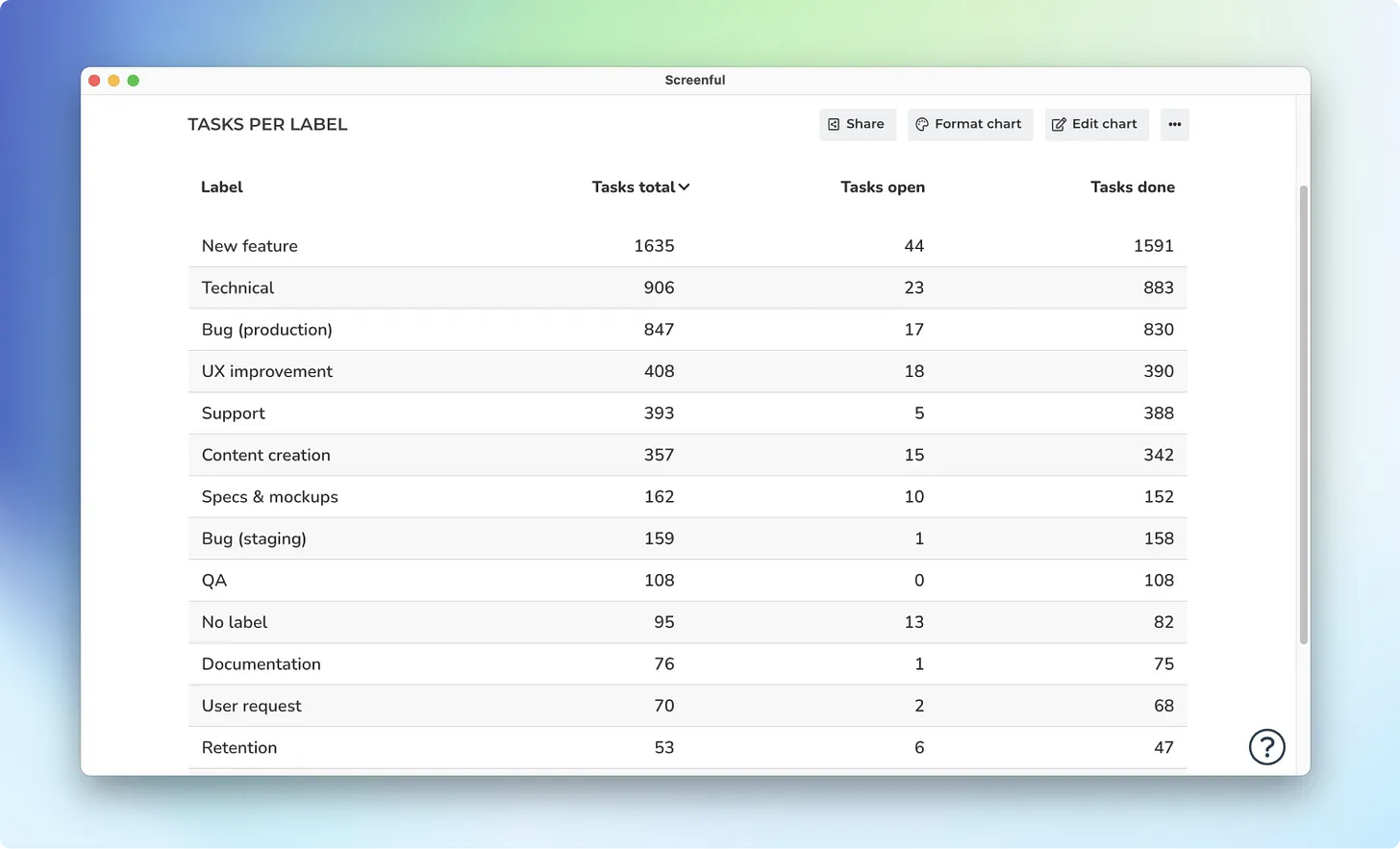It is similar to a Pivot table as it aggregates the task data into sums, averages, and other statistics. It allows to rapidly analyse large amounts of task data in different ways. For example, you can track key metrics such as velocity and cycle times across all of your boards.

What makes this chart really powerful is that you can have as many columns as you want, and each of them can be configured separately.

The calculated column is a special type of column which displays data that is computed based on the data in the other columns. To add a calculated column, click Edit chart and scroll down to the column definitions. Click Add calculated column.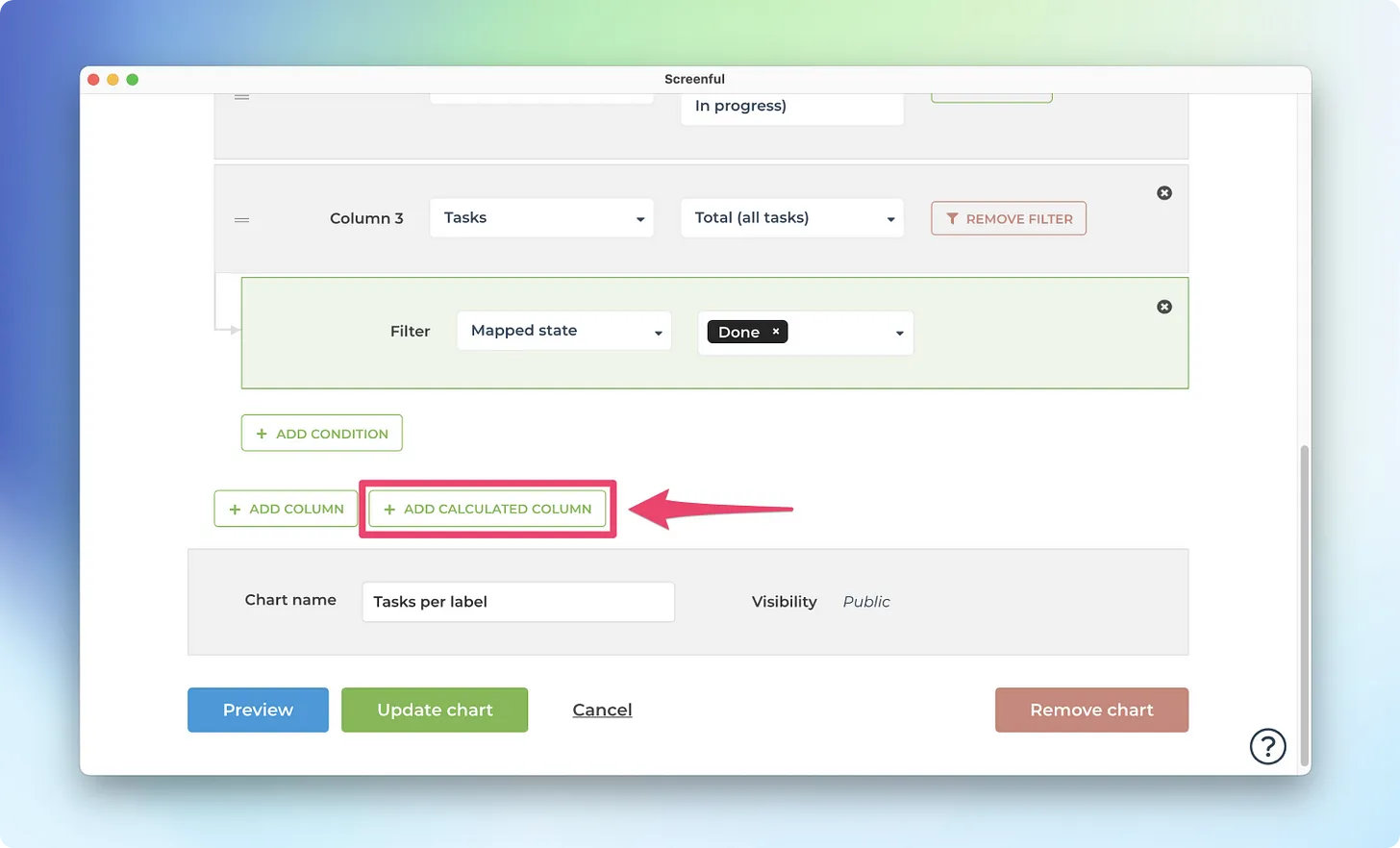A modal is opened which allows entering a mathematical formula.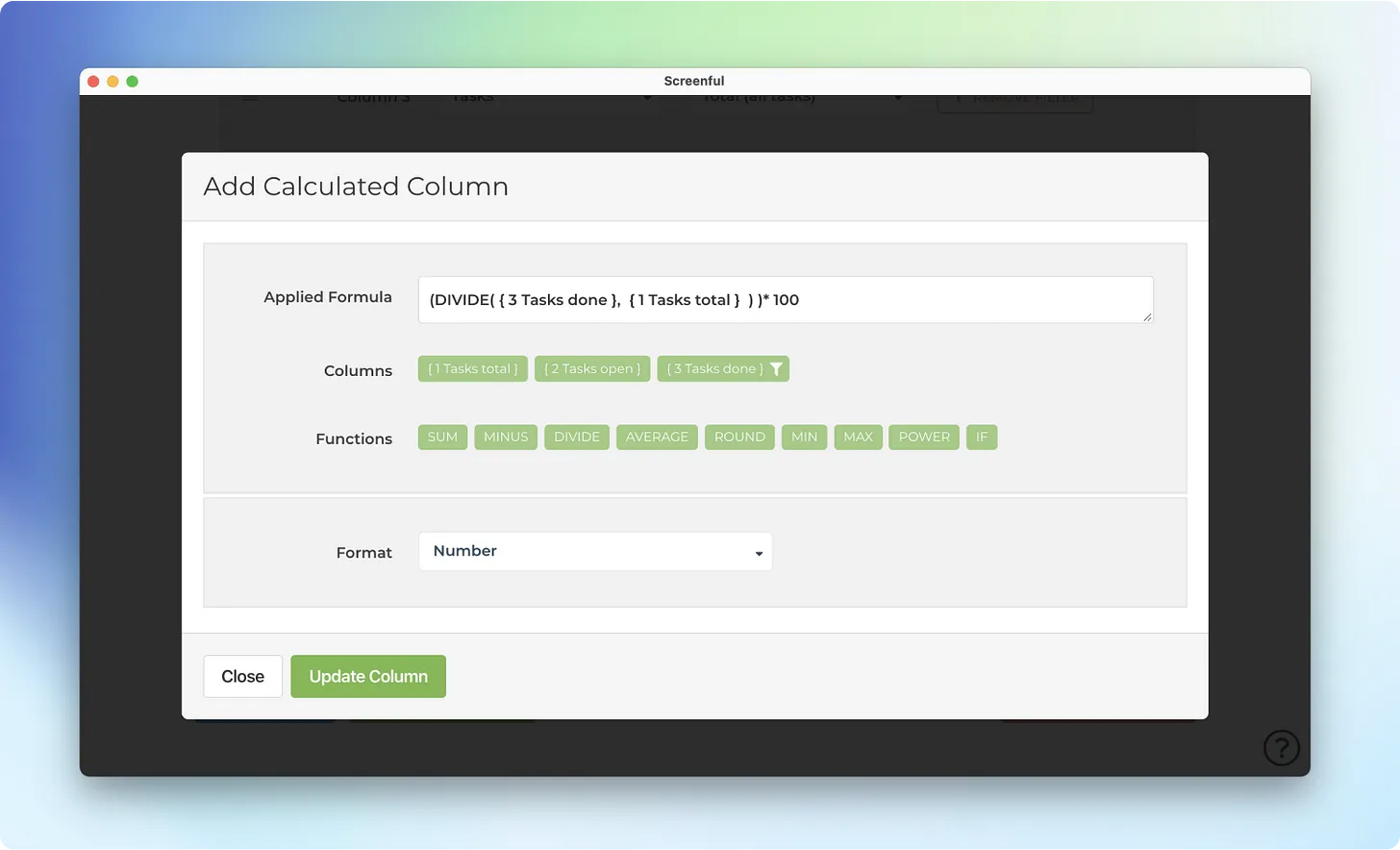In this example, the formula entered is

(DIVIDE( { 3 Tasks done }, { 1 Tasks total } ) )* 100

What it means is that the value in column 3 (Tasks done) is divided by the value in column 1 (Tasks total). The result should show the percentage of work completed.

While it may look complicated, you don’t really have to type it as you can click on the green buttons below the formula field, which provide shortcuts to the most commonly needed formulas. If you’re a power user looking for a more complicated formula, here’s a reference of all the supported formulas.

Once you’ve added the formula and saved the chart, the calculated column is added to the chart: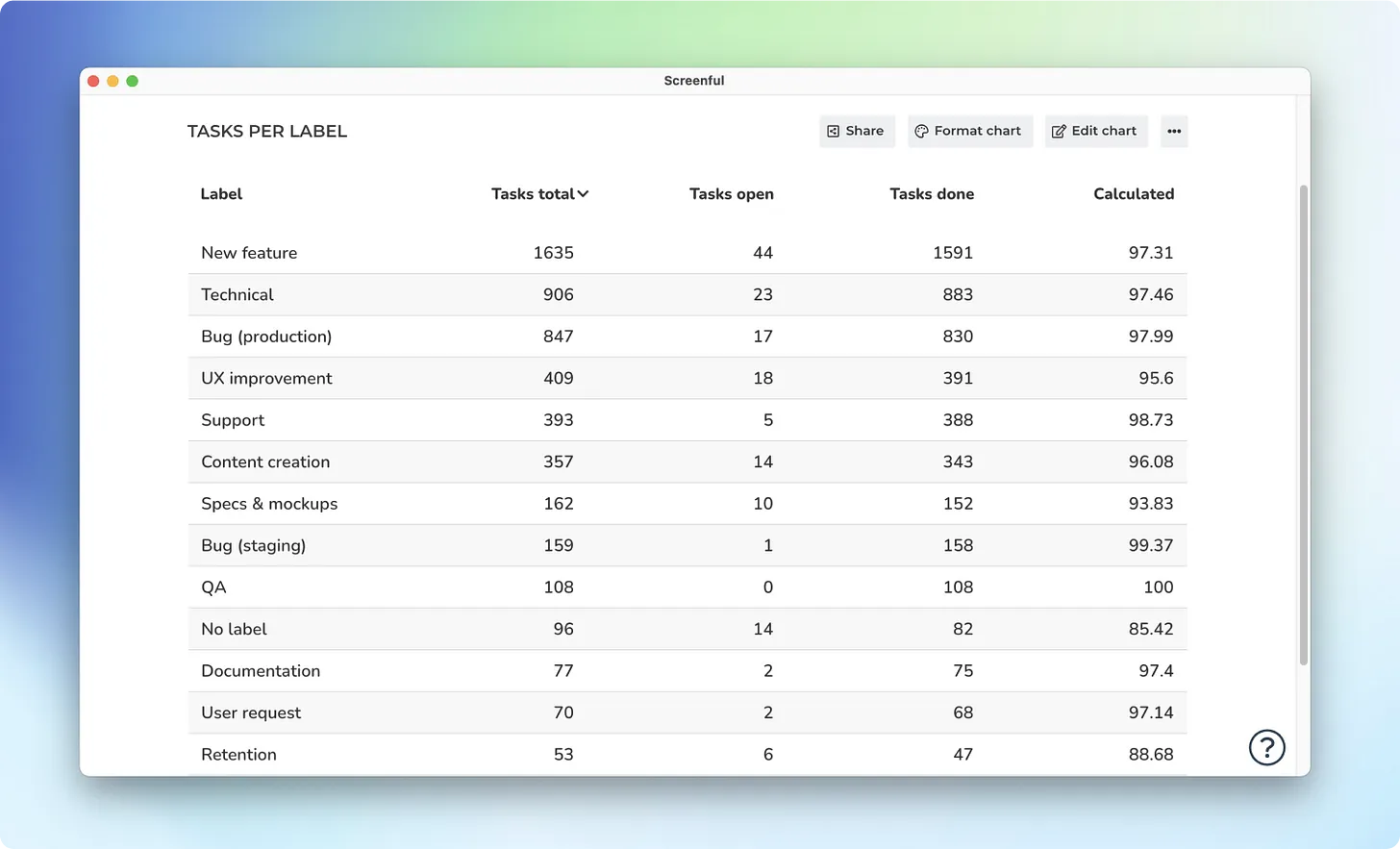Now the rightmost column shows the completion percentage based on the values in the Tasks total and Tasks done columns.

When you write a calculated column formula, it is automatically applied to the whole table and evaluated individually for each row.

## Formatting the calculated column

There are a couple of things you can do to spice up your calculated column. A more visual way of displaying percentages is by using a progress bars. To change the representation, select Progress Bar (0-100) from the Format menu.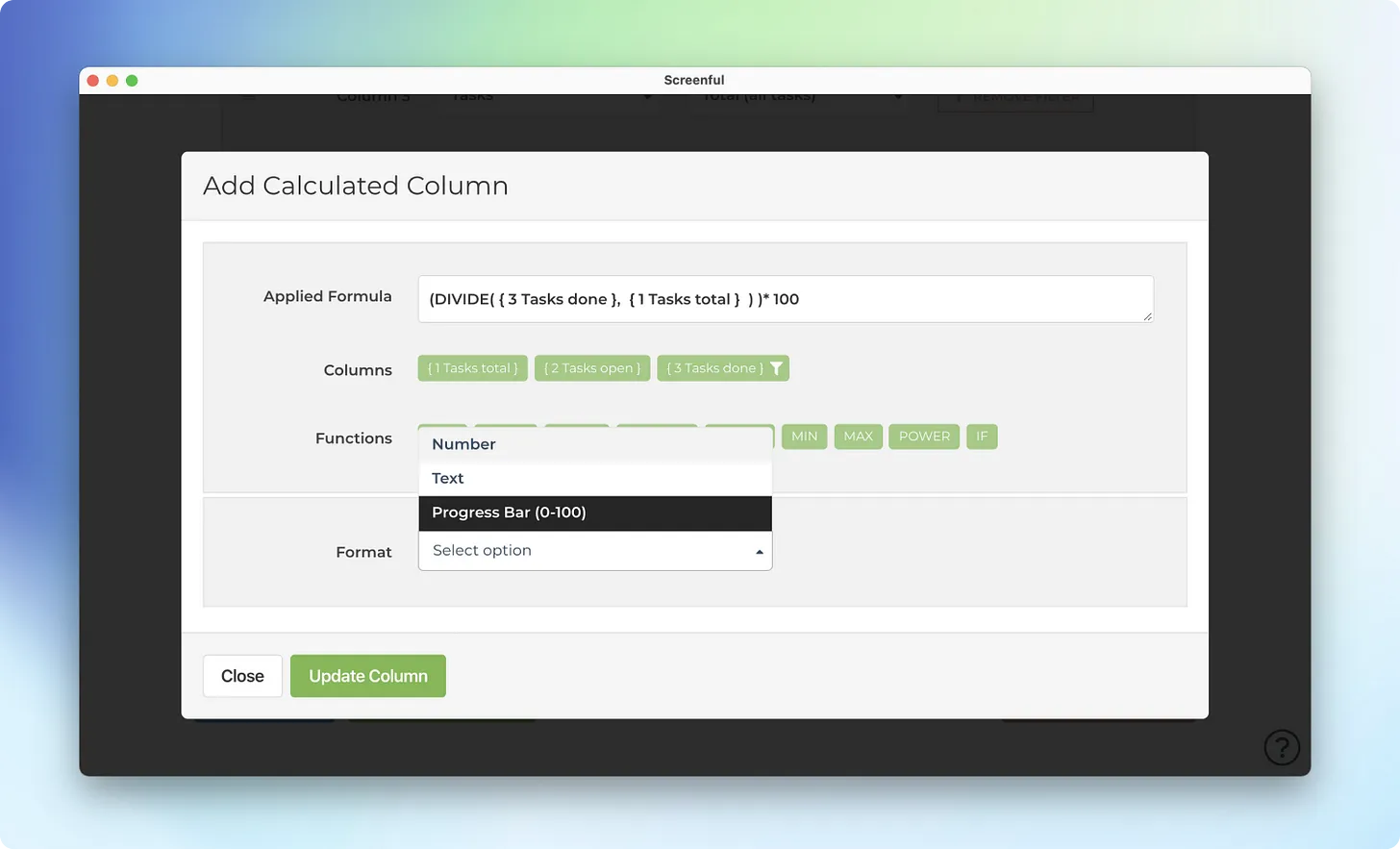You can also change the column header from the default “Calculated” to a more descriptive one in the Chart format section.

Finally, you can reorder the columns by drag and drop. In the below example, we’ve renamed the column header to Progress, and placed it as the first column in the chart. Here’s the final chart: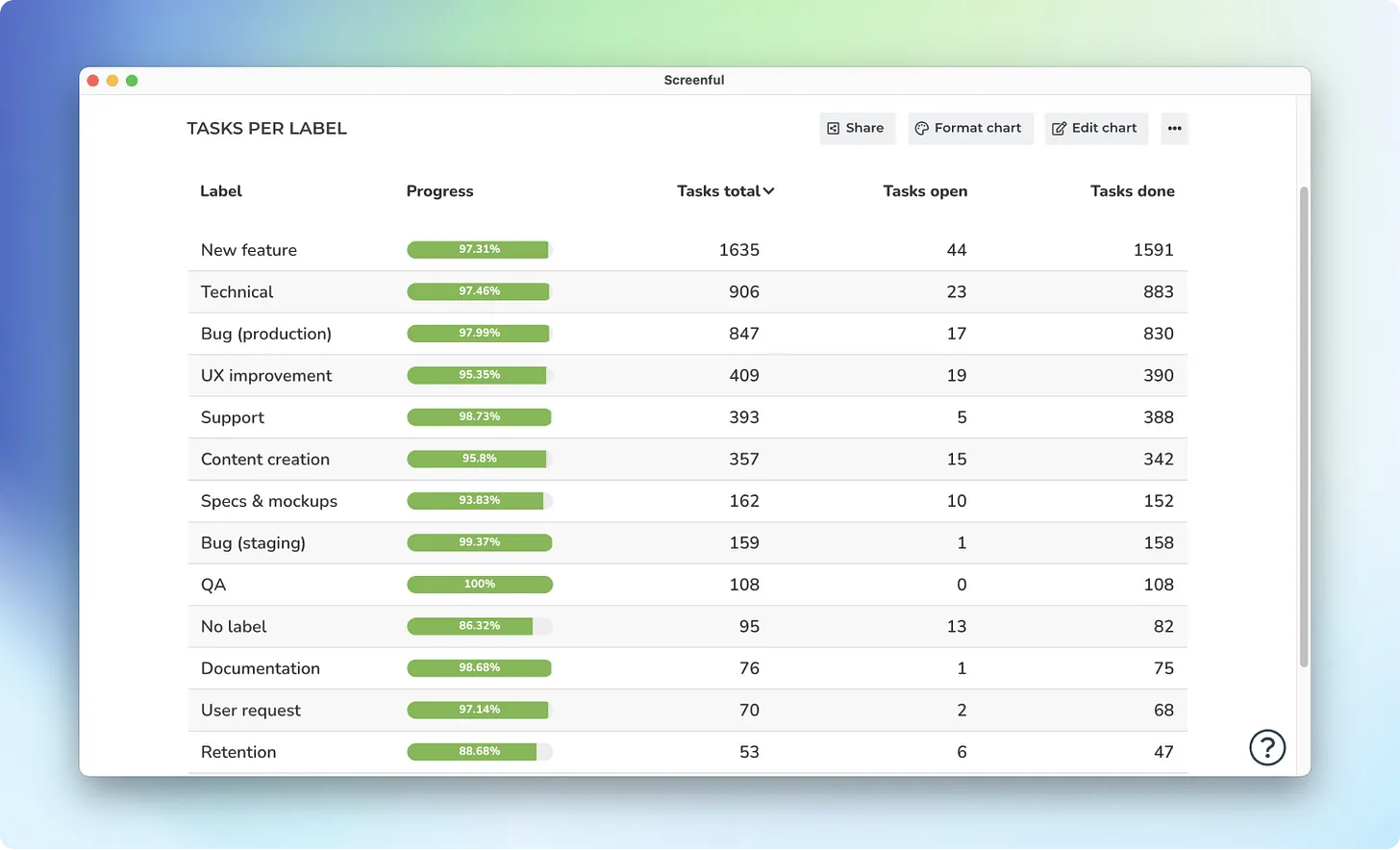## Summary

Table chart is now available for all users. With the Table chart you can create progress lists for your projects, epics, team members, or whatever it is that you want to track. With configurable columns and support for formulas, it allows great flexibility.

We have added a new chart type into the library of available custom charts. The new Table chart allows to view data in rows and columns. You can choose how your data is grouped into rows, and you can configure the columns to display exactly the information you need.

Here’s an example of a Table chart:It is similar to a Pivot table as it aggregates the task data into sums, averages, and other statistics. It allows to rapidly analyse large amounts of task data in different ways. For example, you can track key metrics such as velocity and cycle times across all of your boards.

What makes this chart really powerful is that you can have as many columns as you want, and each of them can be configured separately.

The calculated column is a special type of column which displays data that is computed based on the data in the other columns. To add a calculated column, click Edit chart and scroll down to the column definitions. Click Add calculated column.A modal is opened which allows entering a mathematical formula.In this example, the formula entered is

(DIVIDE( { 3 Tasks done }, { 1 Tasks total } ) )* 100

What it means is that the value in column 3 (Tasks done) is divided by the value in column 1 (Tasks total). The result should show the percentage of work completed.

While it may look complicated, you don’t really have to type it as you can click on the green buttons below the formula field, which provide shortcuts to the most commonly needed formulas. If you’re a power user looking for a more complicated formula, here’s a reference of all the supported formulas.

Once you’ve added the formula and saved the chart, the calculated column is added to the chart:Now the rightmost column shows the completion percentage based on the values in the Tasks total and Tasks done columns.

When you write a calculated column formula, it is automatically applied to the whole table and evaluated individually for each row.

## Formatting the calculated column

There are a couple of things you can do to spice up your calculated column. A more visual way of displaying percentages is by using a progress bars. To change the representation, select Progress Bar (0-100) from the Format menu.You can also change the column header from the default “Calculated” to a more descriptive one in the Chart format section.

Finally, you can reorder the columns by drag and drop. In the below example, we’ve renamed the column header to Progress, and placed it as the first column in the chart. Here’s the final chart:## Summary

Table chart is now available for all users. With the Table chart you can create progress lists for your projects, epics, team members, or whatever it is that you want to track. With configurable columns and support for formulas, it allows great flexibility.

We have added a new chart type into the library of available custom charts. The new Table chart allows to view data in rows and columns. You can choose how your data is grouped into rows, and you can configure the columns to display exactly the information you need.

Here’s an example of a Table chart:It is similar to a Pivot table as it aggregates the task data into sums, averages, and other statistics. It allows to rapidly analyse large amounts of task data in different ways. For example, you can track key metrics such as velocity and cycle times across all of your boards.

What makes this chart really powerful is that you can have as many columns as you want, and each of them can be configured separately.

The calculated column is a special type of column which displays data that is computed based on the data in the other columns. To add a calculated column, click Edit chart and scroll down to the column definitions. Click Add calculated column.A modal is opened which allows entering a mathematical formula.In this example, the formula entered is

(DIVIDE( { 3 Tasks done }, { 1 Tasks total } ) )* 100

What it means is that the value in column 3 (Tasks done) is divided by the value in column 1 (Tasks total). The result should show the percentage of work completed.

While it may look complicated, you don’t really have to type it as you can click on the green buttons below the formula field, which provide shortcuts to the most commonly needed formulas. If you’re a power user looking for a more complicated formula, here’s a reference of all the supported formulas.

Once you’ve added the formula and saved the chart, the calculated column is added to the chart:Now the rightmost column shows the completion percentage based on the values in the Tasks total and Tasks done columns.

When you write a calculated column formula, it is automatically applied to the whole table and evaluated individually for each row.

## Formatting the calculated column

There are a couple of things you can do to spice up your calculated column. A more visual way of displaying percentages is by using a progress bars. To change the representation, select Progress Bar (0-100) from the Format menu.You can also change the column header from the default “Calculated” to a more descriptive one in the Chart format section.

Finally, you can reorder the columns by drag and drop. In the below example, we’ve renamed the column header to Progress, and placed it as the first column in the chart. Here’s the final chart:## Summary

Table chart is now available for all users. With the Table chart you can create progress lists for your projects, epics, team members, or whatever it is that you want to track. With configurable columns and support for formulas, it allows great flexibility.

Let us know if you have questions or feedback by contacting hello@screenful.com. To stay on the loop, read our blog, or follow us on LinkedIn